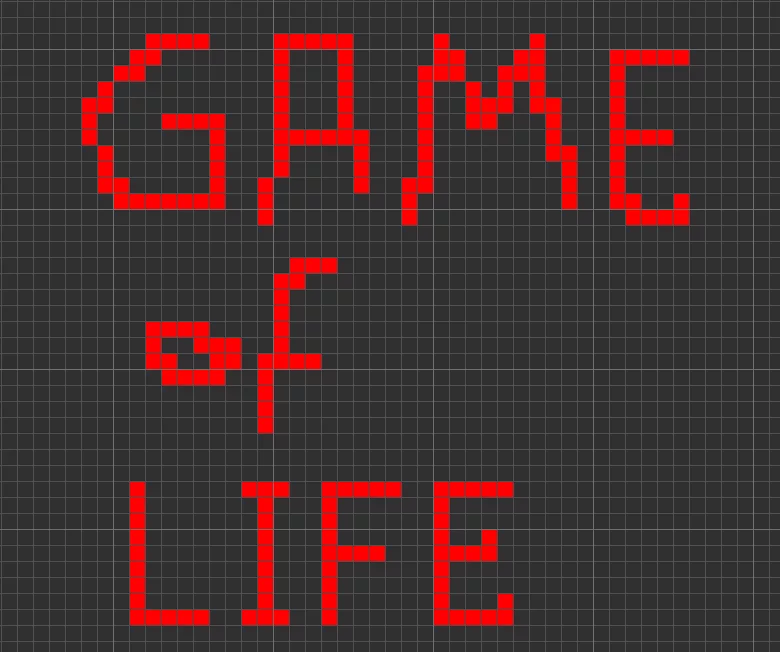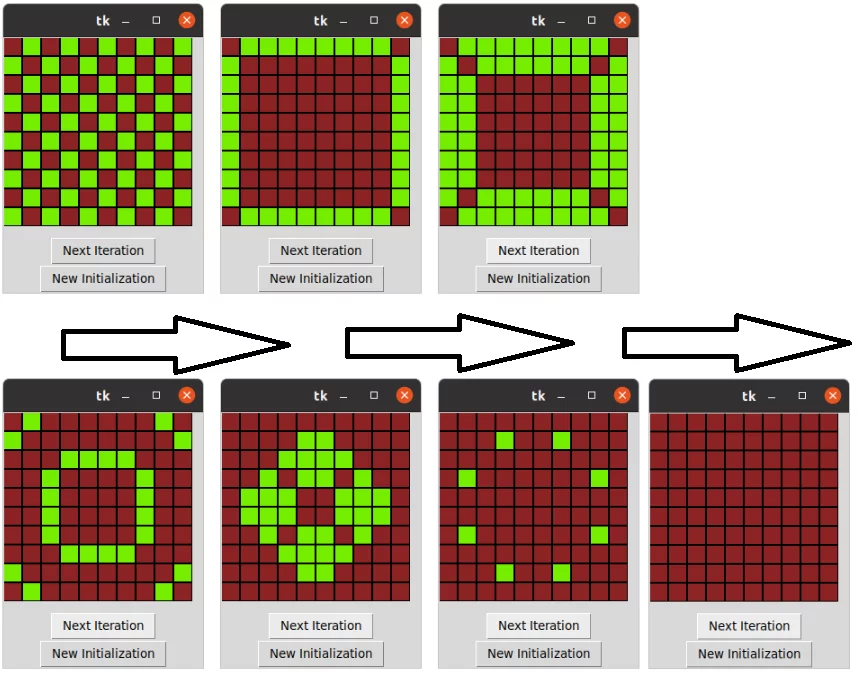## The Game of Life

### IntroductionThe Game of Life, a well-known a zero player game, is introduced by John Horton Conway in 1970. The game is all about the evolution of cells, and the player merely enjoys observing several stages of it (and maybe even programming it in your case :-) ). Imagine the cells as two dimensional orthogonal square cells – and their neighbours as the cells that are either horizontally or vertically or diagonally adjacent. In each evolution phase the following rules apply:

"1- Any live cell with fewer than two live neighbours dies, as if by underpopulation.

2- Any live cell with two or three live neighbours lives on to the next generation.

3-Any live cell with more than three live neighbours dies, as if by overpopulation.

4-Any dead cell with exactly three live neighbours becomes a live cell, as if by reproduction."*

Depending on how you decide to mark the cells as dead or alive, i.e 50% being dead and 50% being alive, you can observe very different patterns. In this short article we’ll show how we programmed “The Game of Life” from the very beginning, which can help you improve yourself further on nested lists and Tkinter.

### Requirements

In order to enjoy this to the most, and be able to observe the beautiful patterns, please make sure you installed Tkinter. Please import the following in the very beginning:

from dataclasses import dataclass
import tkinter
from random import random
import sys

if sys.version_info.major == 3 and sys.version_info.minor >= 9:
listtype = list
else:
from typing import List
listtype = List


Due to some differences between Python 3.9 and 3.8, we've decided to add the following statement. In case you have Python 3.9, "list" is fine, however if you don't then you need to import List from typing to be able to use it in the way we did. So we imported all those to make sure it runs on all versions of Python 3.

### Creating the Cells

The most important element of this game are without doubt, the cells. We are going to change their status according to their neighbours, that’s why we created a class Cell, with the attributes value andmarked. Value is an integer, which will be either 0 for dead cells, or 1 for alive cells. It’s going to help us calculate the neighbours of a cell. Marked is a boolean, which is set to False as default, but if the cell is alive, it will be set to true. This attribute will help us update the board after checking the number of living neighbours.

We used a decorator @dataclass to make this kind of a class possible. You'll find more information on this in a following chapter.

@dataclass
class Cell:
value: int
marked: bool = False

boardtype = listtype[listtype[Cell]]


### Creating the board

To keep the things easy, we created a 10 * 10 board. If you prefer a larger board, feel free to try it out! Our board is going to be a two dimensional list, that’s why we used two for loops.

You can also use other board initializations but this one gives you a board filled with 1s and0s due to the %2. And 1s and 0s are what we are going to need in the Game of Life.

board = list()
for i in range(10):
board.append(list())
for j in range(10):
board[-1].append(Cell((i+j) % 2))


### Random Initializer

The next step about the board is randomly spreading the live / dead cells. That’s why we imported random from random import random we need a random initializer so that we can discover different shapes and patterns in each try. In the following code we made sure that we made half of the cells live. Feel free to play with this value (0.5) to create different random patterns.

def random_init(board:boardtype) -> None:
for i in range(len(board)):
for j in range(len(board)):
a = random()
board[i][j].marked = False
if a < 0.5:
board[i][j].value = 0
else:
board[i][j].value = 1


### Updating Field

We need to make sure that we mark the dead and live cells with different colors. We chose green forlive cells and brown for the dead ones. Feel free to discover Tkinter’s color library to choose different colors.

def update_field(f: tkinter.Canvas) -> None:
for i in range(len(board)):
for j in range(len(board)):
if board[i][j].value == 1:
field.create_rectangle(i*21, j*21, i*21 + 20,j*21 + 20, fill='chartreuse2')
else:
field.create_rectangle(i*21, j*21, i*21 + 20, j*21+20, fill='brown4')


root = tkinter.Tk()
field = tkinter.Canvas(root, width=220,height=220)
field.pack()
next_button = tkinter.Button(root, text="Next Iteration", command=lambda : run_and_canvas(board, field))
next_button.pack()
init_button = tkinter.Button(root, text="New Initialization", command=lambda : random_init_tk(board, field))
init_button.pack()
tkinter.mainloop()


and lastly it is tkinter.mainloop() that makes the whole simulation run.

### Creating the algorithm

Now that our board is ready, we’ll create the algorithm. We need some helping functions for calculating the number of neighbours, checking all cells, and updating the board accordingly.

### Calculating the number of neighbours and evading the list index out of range!

A random square in a grid may have max. 8 neighbours, 3 on the top, 2 on the side and 3 at the bottom. We need the number of alive cells so let’s make it a variable and set it to 0. Whenever we find a live cell, we’ll increment it by 1. We use the following case for the squares which are not on the edges.

In [ ]:
elif row != len(board)-1 and col != len(board)-1:
if board[row-1][col-1].value == 1:
alive_cells += 1
if board[row-1][col].value == 1:
alive_cells += 1
if board[row-1][col+1].value == 1:
alive_cells += 1
if board[row][col-1].value == 1:
alive_cells += 1
if board[row][col+1].value == 1:
alive_cells += 1
if board[row+1][col-1].value == 1:
alive_cells += 1
if board[row+1][col].value == 1:
alive_cells += 1
if board[row+1][col+1].value == 1:
alive_cells += 1


However, what if we check the neighbours of the cells that are at the edges of the board? This code cannot be applied to them, it’d throw a list index out of range error. We have different scenarios:

#### 3 neighbours:

The cell can also be at the top left (0,0), we could avoid the list index out of range error by:

In [ ]:
if row == 0 and col == 0:
if board[row][col+1].value == 1:
alive_cells += 1
if board[row+1][col+1].value == 1:
alive_cells += 1
if board[row+1][col].value == 1:
alive_cells += 1


Or top right (0,9), we could avoid the list index out of range error by:

In [ ]:
elif row == 0 and col==len(board)-1:
if board[row][col-1].value == 1:
alive_cells += 1
if board[row-1][col-1].value == 1:
alive_cells += 1
if board[row-1][col].value == 1:
alive_cells += 1


The cell can also be at the bottom left (9,0), we could avoid the list index out of range error by:

In [ ]:
elif row == len(board)-1 and col==0:
if board[row-1][col].value == 1:
alive_cells += 1
if board[row-1][col+1].value == 1:
alive_cells += 1
if board[row][col+1].value == 1:
alive_cells += 1


Or bottom right (9.9), we could avoid the list index out of range error by:

In [ ]:
elif row == len(board)-1 and col == len(board)-1:
if board[row-1][col].value == 1:
alive_cells += 1
if board[row-1][col-1].value == 1:
alive_cells += 1
if board[row][col-1].value == 1:
alive_cells += 1


#### 5 neighbours:

The cell can be on the top, we could avoid the list index out of range error by:

In [ ]:
elif row == 0 and col !=0 and col !=len(board)-1:
if board[row][col-1].value == 1:
alive_cells += 1
if board[row][col+1].value == 1:
alive_cells += 1
if board[row+1][col-1].value == 1:
alive_cells += 1
if board[row+1][col].value == 1:
alive_cells += 1
if board[row+1][col+1].value == 1:
alive_cells += 1


The cell can be on the left end, we could avoid the list index out of range error by:

In [ ]:
elif row != 0 and row!= len(board)-1 and col == 0:
if board[row-1][col].value == 1:
alive_cells += 1
if board[row-1][col+1].value == 1:
alive_cells += 1
if board[row][col+1].value == 1:
alive_cells += 1
if board[row+1][col].value == 1:
alive_cells += 1
if board[row+1][col+1].value == 1:
alive_cells += 1


The cell can be on the right end, we could avoid the list index out of range error by:

In [ ]:
elif row != len(board)-1 and row != 0 and col == len(board)-1:
if board[row-1][col].value == 1:
alive_cells += 1
if board[row-1][col-1].value == 1:
alive_cells += 1
if board[row][col-1].value == 1:
alive_cells += 1
if board[row+1][col-1].value == 1:
alive_cells += 1
if board[row+1][col].value == 1:
alive_cells += 1


The cell can be at the bottom, we could avoid the list index out of range error by:

In [ ]:
elif row == len(board)-1 and col != len(board)-1 and col!= 0:
if board[row][col+1].value == 1:
alive_cells += 1
if board[row][col-1].value == 1:
alive_cells += 1
if board[row-1][col+1].value == 1:
alive_cells += 1
if board[row-1][col].value == 1:
alive_cells += 1
if board[row-1][col-1].value == 1:
alive_cells += 1


However, as you see this is pretty long and takes a lot of effort, yet we thought that this way of thinking may be helpful in constructing the following code. After all, the codes should make things easy for us.

In [ ]:
def calculate_neighbours(board: list[list[Cell]], row: int, col: int):
neighbours = 0
for i in range(row-1,row+2):
for j in range(col-1, col+2):
if i >= 0 and j >= 0 and i < len(board) and j < len(board):
neighbours += board[i][j].value
neighbours -= board[row][col].value
return neighbours


Here we firstly substract 1 from the given row and column, and if it is smaller than 0, we know that we will get an out of range error. We add +2 to the row and column so that we can check its other neighbours. To avoid the out of range error, we check if the result is smaller than the length of the board for row, and for the column its enough the check the elements of the first row of the board, to find out how many columns there are. If all the criterias are met, we append the value to the neighbours. But in this scenario, we also check the value of our own cell, that’s why after the loop we get rid of it.

### Checking all the cells:

Here we apply the rules about living up to the next generation, which are stated at the beginning. We first change the marks on the cells, which indicate whether they are dead or alive. We don’t change the values immediately because we need the original board to stay the same for now. Else, ifthe value of a cell changes the neighbour will get affected by it, and so will their neighbours and wewould have a completely different scenario at the end, far away from our expectations and the rules mentioned. We will update the values in the upcoming function.

In [ ]:
def check_all_cells(board: list[list[Cell]]) -> None:
for row in range(len(board)):
for col in range(len(board)):
number_of_neighbours = calculate_neighbours(board, row, col)
if board[row][col].value == 1:
if number_of_neighbours > 3:
board[row][col].marked = False
if number_of_neighbours < 2:
board[row][col].marked = False
if number_of_neighbours == 2 or number_of_neighbours ==3:
board[row][col].marked = True
elif board[row][col].value == 0:
if number_of_neighbours == 3:
board[row][col].marked = True
else:board[row][col].marked = False


### Updating the board

In this function we change the values of the marked cells to 1, and the unmarked cells to 0.

In [ ]:
def update_board(board: list[list[Cell]]) -> None:
for row in range(len(board)):
for col in range(len(board)):
if board[row][col].marked == True:
board[row][col].value = 1
else:
board[row][col].value = 0


### Running the functions

We need another function to run these in the wished sequence. We called ours run_and_canvas. This is where Tkinter gets the updates and creates the next generation.

In [ ]:
def run_and_canvas(board: list[list[Cell]], f: tkinter.Canvas) -> None:
check_all_cells(board)
update_board(board)
update_field(f)


We also add sort of a copy of our update field function to the end, to make sure a nice board is there to greet us when we run the program.

In [ ]:
for i in range(len(board)):
for j in range(len(board)):
if board[i][j].value == 1:field.create_rectangle(i*21, j*21, i*21 + 20,j*21 + 20, fill='chartreuse2')
else:
field.create_rectangle(i*21, j*21, i*21 + 20, j*21+20, fill='brown4')


### A Sample SimulationYou can find our code here